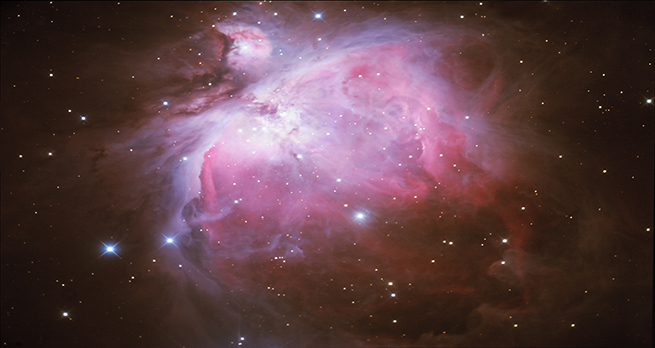In the night sky: Orion

Start this free course now. Just create an account and sign in. Enrol and complete the course for a free statement of participation or digital badge if available.

Free course

# 2.1.3 How far away is the Orion nebula?

How far away is the Orion Nebula? An ‘astronomical’ distance would be a good description!

It is estimated to be about 1600 light years away. It may sound a bit odd, to use time as a measurement of distance, but as Monica explains in the video, that is because the distances are so large. A light year is the distance light travels in one year. We can calculate what that is in kilometres or miles, as you can see in the following video.Skip transcript

#### Transcript

The thing about astronomy is the distances are so enormous it doesn’t really make much sense to talk in terms of miles or kilometres.
So we use a unit called a light-year, now that might sound a bit odd because of course a year is a measure of time and not distance, but let me explain how we do it.
So a year is equal to 365 days, except every 4 years we have a leap year, so it works out on average to be 365.25 days. Now, a 365.25 days has got 24 hours in it, each hour has got 60 minutes in it, each minute has got 60 seconds in it. So actually, one year is this many seconds.
If we change tack about it now and think about how fast light travels, light travels, very fast. It travels at 300 million metres in one second, so if you put that together with how many seconds there are in a year, what you come to is that light travels in one year, it actually travels about 9.5 million million kilometres or 6 million million miles.
So in one year it travels 9.5 million million kilometres or 6 million million miles. The nearest star to us, Proxima Centauri, is four light-years away. So Proxima Centauri is four times 9.5, so that’s 38, 38 million million kilometres away, or 24 million million miles away.
That’s why we think in terms of light-years, because the numbers are much easier to grasp.
End transcript

Interactive feature not available in single page view (see it in standard view).

So light travels about 9.5 million million kilometres, or 6 million million miles in a year.

If the Orion Nebula is 1600 light years away, that is 1600 x 9.5 million million kilometres – the best part of 15.2 thousand million million kilometres or about 9.6 thousand million million miles – which is quite far away. And also explains why astronomers use light years as a unit of distance.